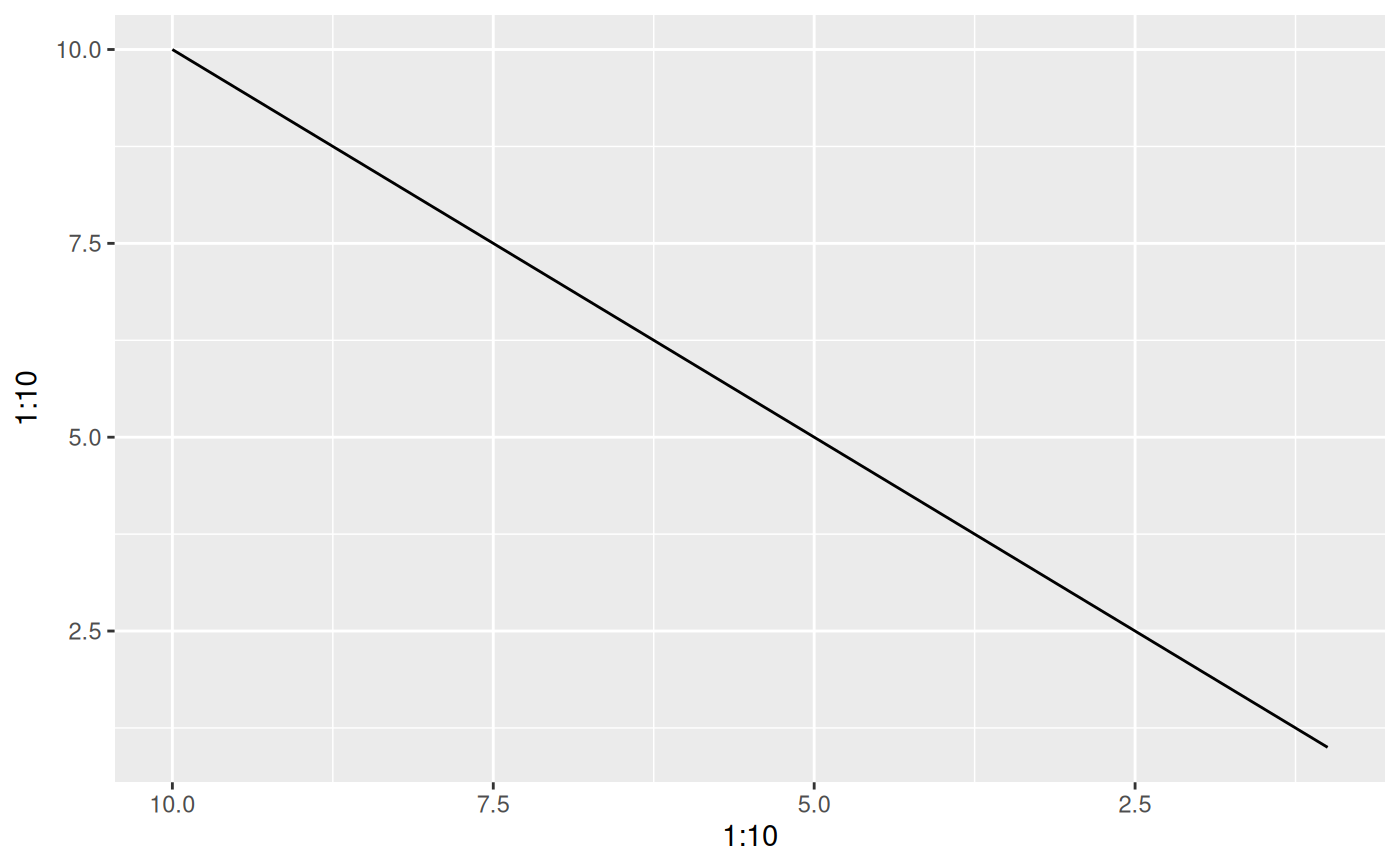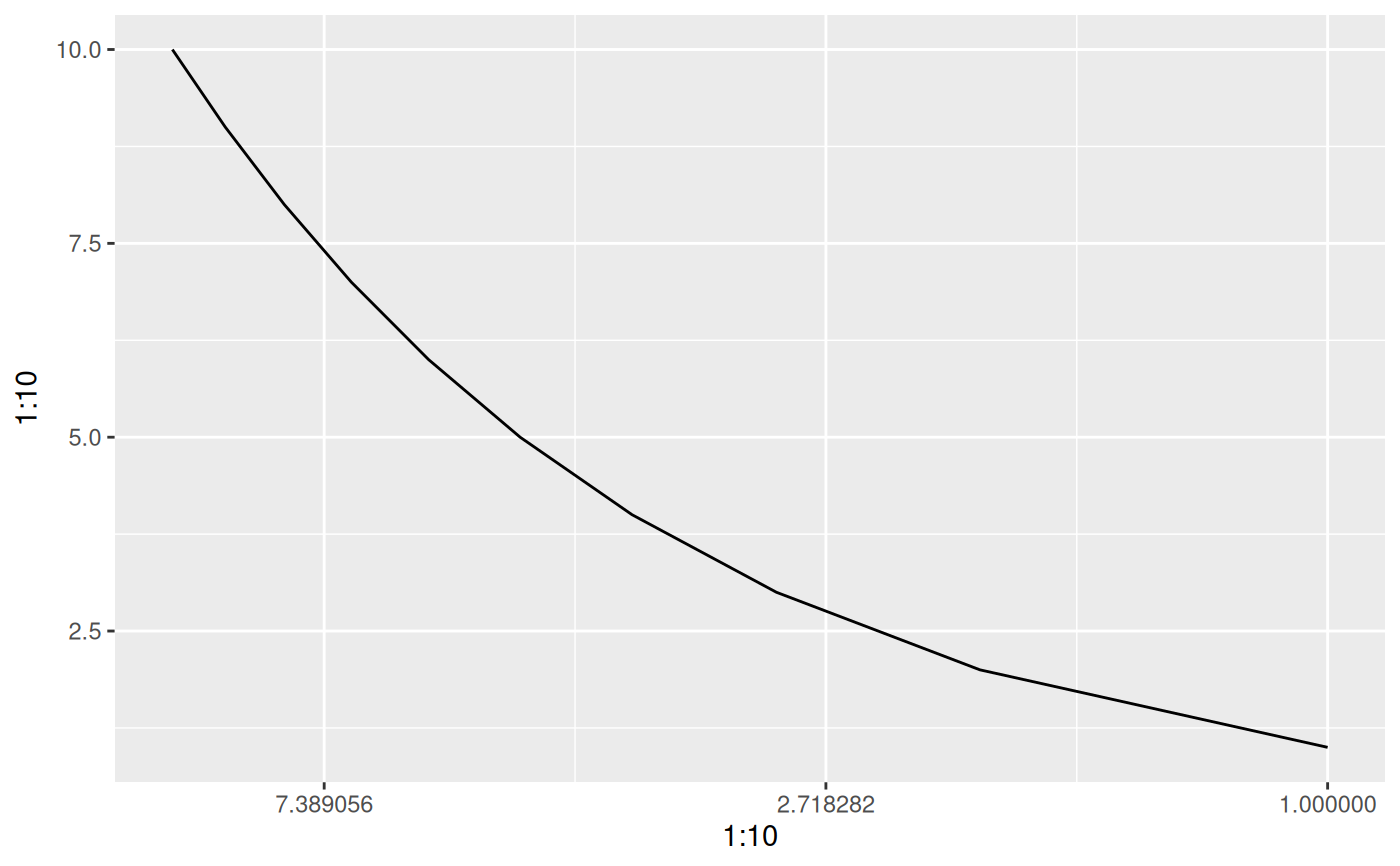While the scales package export a reverse_trans object it does not allow for reversing of already transformed ranged - e.g. a reverse exp transformation is not possible. trans_reverser takes a trans object or something coercible to one and creates a reverse version of it.

trans_reverser(trans)

## Arguments

trans

A trans object or an object that can be converted to one using scales::as.trans()

A trans object

## Examples

# Lets make a plot
p <- ggplot() +
geom_line(aes(x = 1:10, y = 1:10))

# scales already have a reverse trans
p + scale_x_continuous(trans = 'reverse')# But what if you wanted to reverse an already log transformed scale?
p + scale_x_continuous(trans = trans_reverser('log'))